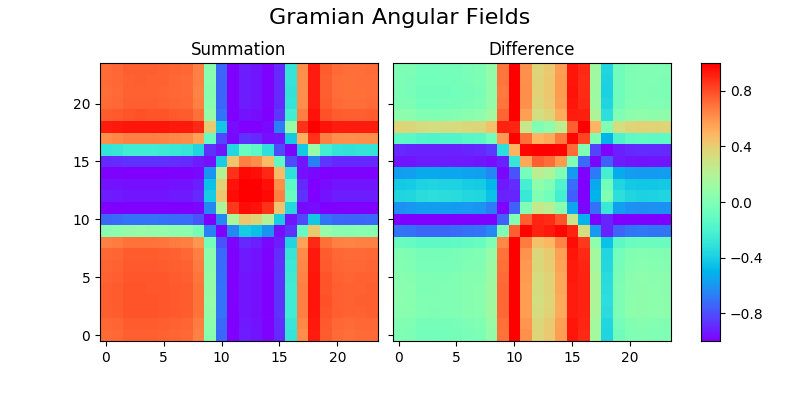# Gramian Angular Field¶

A Gramian Angular Field is an image obtained from a time series, representing some temporal correlation between each time point. Two methods are available: Gramian Angular Summation Field and Gramian Angular Difference Field. This example illustrates the transformation on the first sample of the GunPoint dataset. Both images are plotted side by side to illustrate the differences. It is implemented as `pyts.image.GramianAngularField`.```# Author: Johann Faouzi <johann.faouzi@gmail.com>

import matplotlib.pyplot as plt
from mpl_toolkits.axes_grid1 import ImageGrid
from pyts.image import GramianAngularField

# Parameters
X, _, _, _ = load_gunpoint(return_X_y=True)

# Transform the time series into Gramian Angular Fields
gasf = GramianAngularField(image_size=24, method='summation')
X_gasf = gasf.fit_transform(X)

# Show the images for the first time series
fig = plt.figure(figsize=(8, 4))
grid = ImageGrid(fig, 111,
nrows_ncols=(1, 2),
share_all=True,
cbar_location="right",
cbar_mode="single",
cbar_size="7%",
)